# Zeros of Dirichlet polynomials

Turan showed that if for all sufficiently large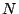, theth partial sum of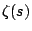does not vanish in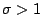then the Riemann Hypothesis follows.

He [ MR 10,286a] strengthened this criterion by showing that for every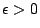there is an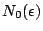such that if theth partial sum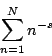of the zeta-function has no zeros in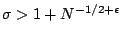for all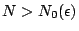then the Riemann Hypothesis holds.

H. Montgomery [ MR 87a:11081] proved that this approach cannot work because for any positive number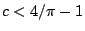theth partial sum ofhas zeros in the half-plane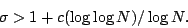Back to the main index for The Riemann Hypothesis.